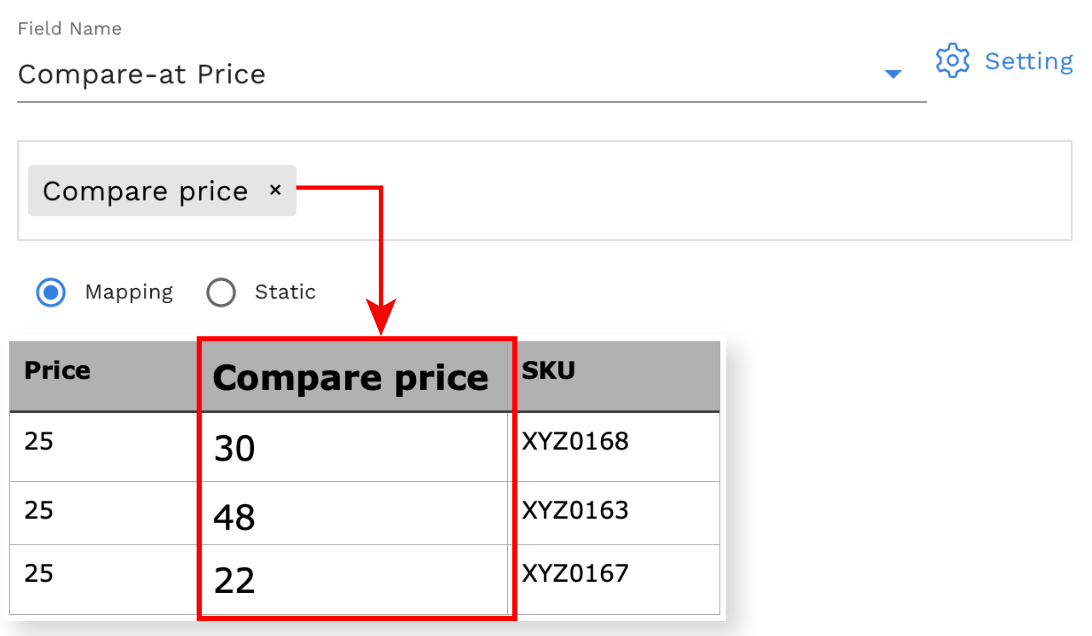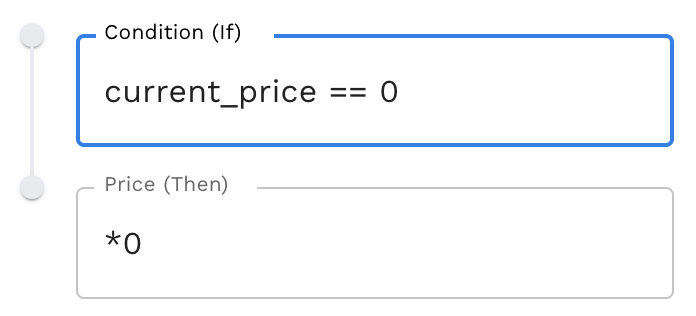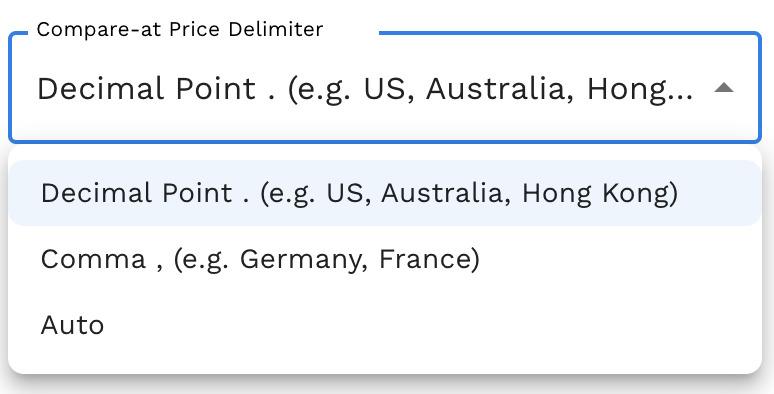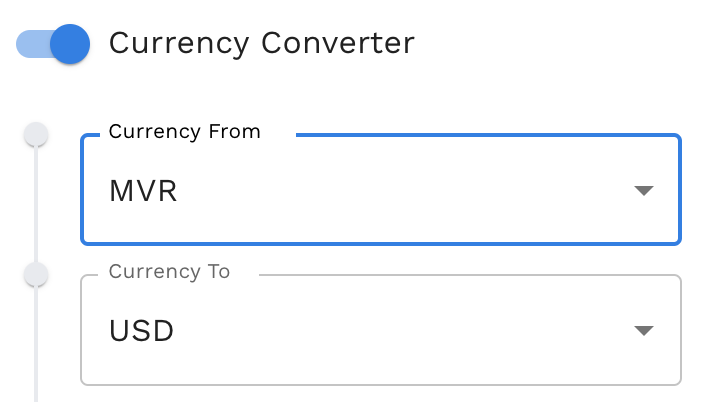# Compare-at Price Field

Modified on Mon, 24 Jul 2023 at 05:00 PM

`Available on: ✔️ Add ✔️ Update ✔️ Export`There are a few settings for this field which are:

1. Compare-at Price Conditions (Markdown / Markup)

How to use Compare-at Price Conditions according to the examples below:`In order to display the compare-at price in the store front, the compare-at price value must be higher than the price. For further information, please refer here.`

 Condition (If) Price (Then) Result Showing current_price == 0 *0 This condition will not update when the price in your store is 0 in the feed file any *1.5 If the price is 11, then the price is multiplied by 1.5 or 50% price_f > 10 and price_f <= 15 *1.2 If the price is more than 10 and equals or below 15, then the price is multiplied by 1.2 or 20%  Use price_f when the comparison values are numeric price_f ==11 1 All the prices will be multiplied by 1.5 or 50% price > col1_f *1 + col1 + col2 If the price is more than column 1, then add column 1 and column 2 to the price. Use col#_f when the comparison values are numeric                                                                                                        Note: col expression is not available for the XML file format col5==“shoe” *1.3 If column 5 has value shoe, then multiply by 1.3This can be used for any column and is suitable if you are planning to add markup based on collections price+f < 1 *0 + current_price If the price is less than 1, then it will use the current price in-store (Shopify only).                                   Use price_f when the comparison values are numeric Price ==“high” *1.2 If the price is equal as "high", then it will multiply by 1.2                                                                  Use price when the comparison values are text any *0.90 All the prices will be multiplied by 0.9 or 10% which means your price will be discounted 10% price ==“discount” *0.50 If the price is equal as "discount", then it will multiply by 0.50 0r 50% which means will markdown the price cost < 40 *1.2 If cost in the store is less than 40, then multiply the price by 1.2. The cost value will be taken from the store. (Shopify only) compare_at_price > 10 *1.5 If compare at price in the store is more than 10, then multiply the price by 1.5. The compare at price value will be taken from the store. (Shopify only) any /0.2 If the col4 date is more than current_timestamp, then multiply by 1.2 col4_date > now *1.2 If the col4 date is more than current_timestamp, then multiply by 1.2                                      Use col4_date when the comparison values are date Now refers to current_timestamp col4_date > today *1.2 If the col4 date is more than current_timestamp, then multiply by 1.2                                      Use col4_date when the comparison values are date  Today refers to today’s date

There is little info regarding the compare-at price condition (markdown / markup)

i. The Price Conditions take effect according to the arrangement. The first conditions have the highest priority. You can move it by using the arrow beside there according to your needs.

ii. Stock Sync now supports Column Value.

iii. Valid price range keywords are:

• price (Use it when the comparison value are text)
• price_f (Use it when the comparison value are numeric)
• col#_f (Use it when the comparison value are numeric)
• any
• current_price (Shopify only)
• cost (Shopify only)
• compare_at_price (Shopify only)

iv. The symbol that is used for the statement:

 Symbol Details == equal to <= less than or equal to >= more than or equal to > more than < less than and only true when both statements are true or true when either statement is true

2. Compare-at Price Delimiter

The price delimiter provided in the price field has three options which are Comma, Decimal Point, and auto.

###3. Compare-at Price Round

A price round is used to standardize the prices of the products in the store if a round number is used too often or the prices do not have a clear focus digit.How to use Compare-at Price Round according to the examples below:

 Round off example Result Showing ##.00 or ##.000 It will change it from 12.57 to 12.00 or 12.573 to 12.000 ##.#0 or ##.#00 Change it from 12.57 to 12.50 or 12.573 to 12.500 ##.## or ##.##0 Change it from 12.57 to 12.57 or 12.573 to 12.570 ##.## or ##.### Change it from 12.57 to 12.57 or 12.573 to 12.573 (Doesn't have any changes) ##.99 Change it from 12.570 to 12.599 ##.95 Change it from 12.57 to 12.95 ##.97 Change it from 12.57 to 12.97 ##.#99 Change it from 12.570 to 12.599 ##9.00 Change it from 121.57 to 129.00 ##5.00 Change it from 123.57 to 125.00 ##9.99 Change it from 127.57 to 129.99 ##,000.00 Change it from 12,453.57 to 12,000.00 ##,#00.00 Change it from 12,345.57 to 12,300.00 ##,##0.00 Change it from 12,346.57 to 12,340.00 ##,#99.00 Change it from 12,345.57 to 12,399.00 ##9 (from #.#9 to ##9,000). eg. 129000, 12900, 1290, 129, 12.9... Change it from 12.57 to 12.90 or 145.67 to 149.00All the third digits of the number will change to 9 followed by 0. nearest whole number (from #1.## to #2.00) Change it from 12.57 to 13.00 or 135.33 to 136.00Every whole number will be rounded up, its meant added by 1

4. Set store value to blank when feed value is blank (value zero is not blank)

By enabling the toggle icon, it will automatically set to blank when the value in the feed file is blank as there is no value set in compare at price section (zero or '0' are not considered as blank).

###5. Currency Converter

If the value of the compare at price provided in the feed is in a different currency than the currency that's in the store.`For example:If the supplier feed currency = MYRAnd the store currency = USDEnable the Currency Converter and enter the currency in the fields above.`

6. Don't update Compare-at price when ...

Exclude certain products from updating the compare at price using this option. It can be excluded by VendorProduct Type (Custom), and Product Tags.```For example:

Dont update Compare-at Price when Vendor = Adibas